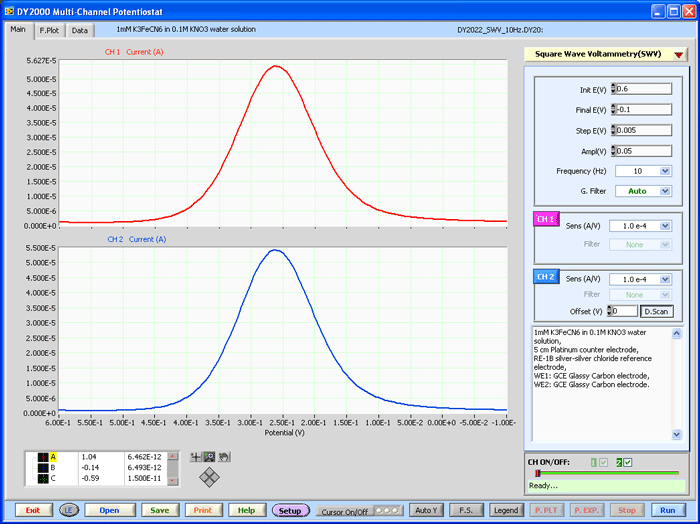## DY2000 Series Multichannel Potentiostat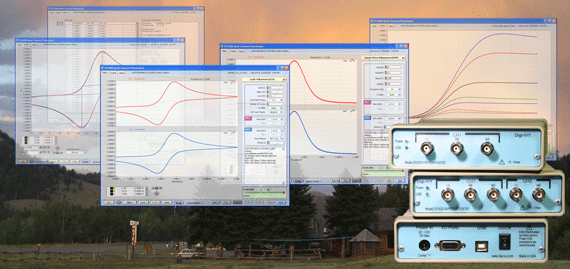## Applications

• Potentiometric and amperometric sensor applications
• Free-radical detection (oxygen, nitric oxide, H2O2, etc.)
• Electrochemical research and education
• Photo-current amplifier
• Multi-channel, high input impedance digitizer
• Many other user-configurable applications

## Hardware

 Max. Output Current ±2.0 mA / CH Controlling Voltage Range ±2.0 V Max. Compliance Voltage ±2.2 V Current Range ±2.0 nA to ±2.0 mA in 7 steps Current Resolution 0.002 % of current range, 76 fA for ±2.0 nA range Current Accuracy < 1.0% of current range Input Bias Current @ 25°C < 20 pA Min. Potential Step (by user) 1.0 mV (60 µV for the hardware) ADC Converter 16-bit, 100 kHz DAC Converter 16-bit Input Channels 1 or 2 depending on hardware configuration Electrode Configuration CH1(CE, RE and WE), CH2 (EC, WE) System Bandwidth > 30 kHz Min. Rise time of Applied Waveform < 2.0 µsec / V Min. Time Base 0.1 msec Max. Data Points per CH 15000 Computer Interface USB with PCs running Windows Dimensions (W x D x H) & Weight 15 x 26 x 5 cm, 1 kg Power Requirements 90-240 VAC, 3 W

## Software Techniques

 Amperometric i-t curve (iT) Sampling Time (sec) = [0.0001 to 10] Cyclic Voltammetry (CV) Scan Rate (V/sec) = [0.001 to 10] Open circuit potential vs. time (OCP) Sampling Time (sec) = [0.0001 to 10] Differential Pulse Voltammetry (DPV) Step E (V) = [0.001 to 0.1], Amplitude (V) = [0.001 to 0.5], Pulse Period (sec) = [0.02 to 100] Normal Pulse Voltammetry (NPV) Step E (V) = [0.001 to 0.5], Pulse Period (sec) = [0.02 to 100] Multi-Step Potential (MSP) Step E (V) = [-2.0 to +2.0], Step Width (sec) = [0.005 to 200] Square Wave Voltammetry (SWV) Step E (V) = [0.001 to 0.1], Frequency (Hz) = [0.01 to 50]

## Sample Plots

•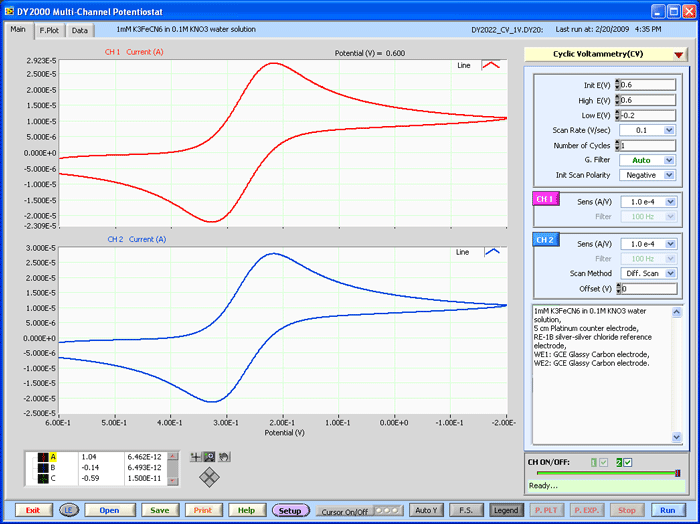•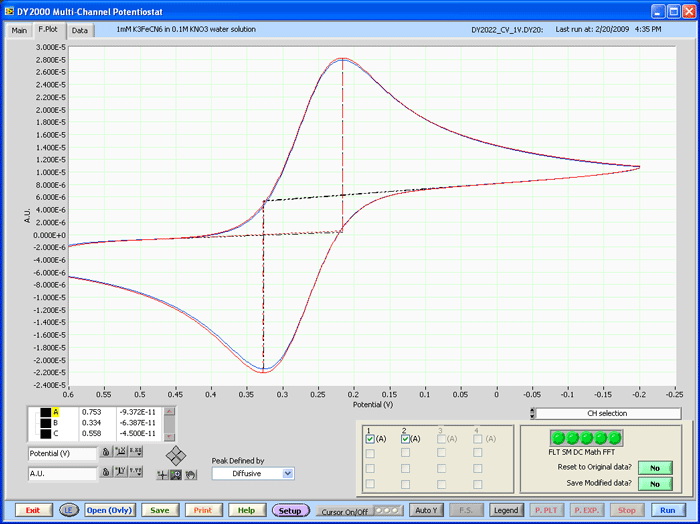•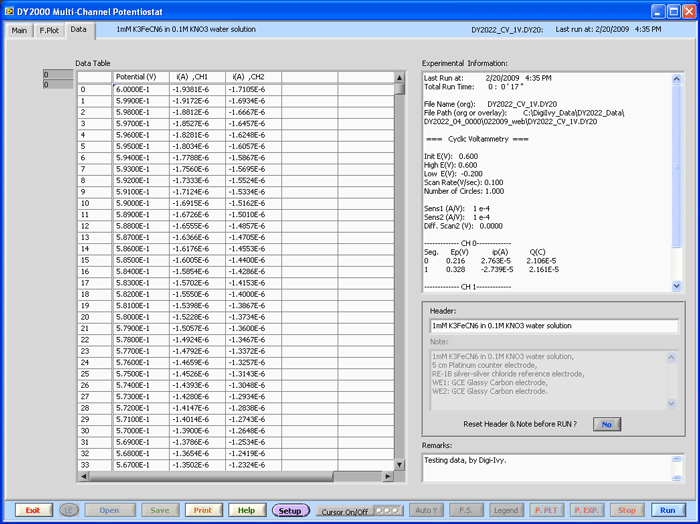•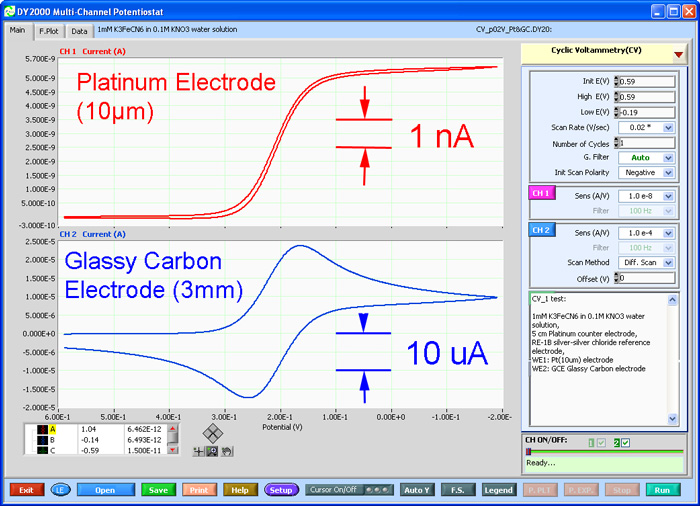•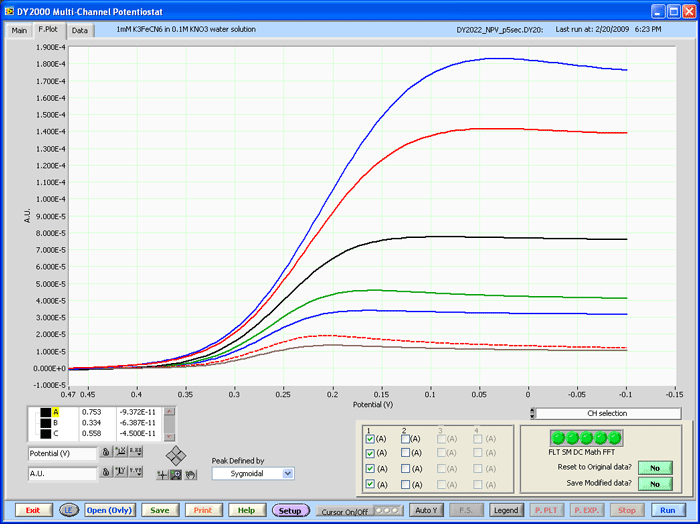•